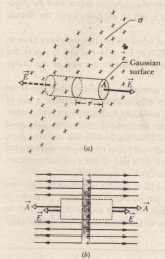# Applying Gauss’ Law: Planar Symmetry

Applying Gauss’ Law:
Planar Symmetry

Nonconducting Sheet

a portion of a thin, infinite, nonconducting sheet with a uniform (positive) surface charge density T A sheet of thin plastic wrap union charged on one side can serve as a simple model Let us find the electric field E a distancer in front of the sheet-A useful Gaussian surface is a closed cylinder with end caps  area A arranged to pierce the sheet perpendicularly as shown From symmetry E must be perpendicular to the sheet and hence to the end caps Furthermore since the charge is positive £ is directed away from the sheet and thus the electric field lines pierce the two Gaussian end caps in an outward direction Because the field lines do not pierce the curved surface there is no flux through this portion of the Gaussian.where is the charge enclosed by the Gaussian surface This gives.

Since we are considering an infinite sheet with uniform charge density this result holds for any point at a finite distance from the sheet agrees with which we found by integration of the electric field components that are
produced by individual charges Look back to that time-consuming and challenging integration and note how much more easily we obtain the result with Gauss’ law That is one reason for devoting a whole chapter to that law for Celina symmetric arrangements of charge. it is very much easier to use than integration of field components.perspective view (a) and side view (b) of a portion of a very large thin plastic sheet. uniformly charged on one side to surface charge density a
A closed cylindrical Gaussian surface passes through the sheet and is perpendicular to.